# The COUNTIFS Function in Excel

COUNTIFS is a powerful statistical function that forms part of the wider Excel Statistical functions category. If you've used the COUNTIF function before, then you're going to love COUTIFS. COUNTIFS can return cells that meet single or multiple criteria.

Where COUNTIF looks at a single condition in one range, COUNTIFS can take into account multiple ranges. It's COUNTIF on steroids!

The COUNTIFS function basically counts cells that could either be in the same or different ranges. It's particularly useful in financial analysis and helps solve a wide range of general Excel dilemmas.

In short, COUNTIFS is an excellent productivity function that makes Excel easier to use. This article will teach you how to put it to work.

• Understanding the COUNTIFS Syntax
• How to Use the COUNTIFS Function in Excel?
• Important Notes About COUNTIFS Function
• Why You’ll Love COUNTIFS
• Get Started With COUNTIFS
• Frequently Asked Questions

## Understanding the COUNTIFS Syntax

Knowing how the COUNTIFS function works will make it easier to analyze data. The first step towards this is understanding the COUNTIFS function syntax:

=COUNTIFS (range1, criteria1, [range2], [criteria2], ...)

Here are the arguments this function uses;

• Range1 - This is the first range of data you wish to evaluate. It is a required argument.
• Criteria1 - The criteria you are looking to count in range1 (also a required argument).
• Range2 - This is the second range of data you wish to evaluate. It is an optional argument.
• Criteria2 - The criteria you are looking to count in range2 (also a required argument).

The criteria can be:

• A numerical value, a decimal, a time, an integer, a date, etc.

Or

• A text string that can include wildcards like an asterisk or question mark.

Fun fact: One single COUNTIFS function can hold up to a maximum of 127 pairs of criteria and ranges!

IMPORTANT: It is vital that the length of the ranges are the same for each argument. If they are not, the function will return a #VALUE! Error.

## How to Use the COUNTIFS Function in Excel?

So, how do we actually use the COUNTIFS function in Excel? COUNTIFS is like any other function in Excel, and because of its flexibility, you can use it to test as many or as few conditions as you need.

Here are some examples to help you understand how COUNTIFS works;

### Example 1 - Using the COUNTIFS Function With Multiple Criteria

Here are the results of students in a class. We want to use the COUNTIFS function to find the students who performed well in this test. In this instance we need to use the COUNTIFS function to find students who got a ‘Pass’ result and achieved  'First’ division marks.COUNTIFS will return the total number of students who scored both a ‘Pass’ result and who achieved a 'First'.As you can see above, Excel has counted the number of students who have met both of these criteria, and has returned the number 3.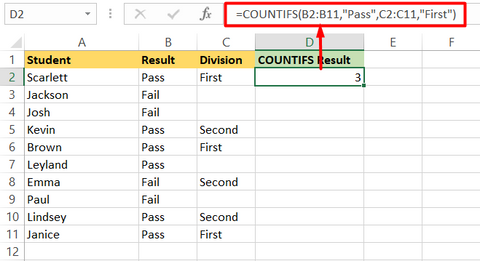### Example 2 - Using COUNTIFS Function With the Same Criteria

You may also want to find the number of students who scored more than 70 marks in two different exams. The table below demonstrates how to find that result using COUNTIFS.In this example, we are adding multiple ranges to our formula and keeping the criteria >70 (greater than 70) for both ranges. Excel is looking at both ranges, and ONLY counting the students that scored 70 or more in both exams.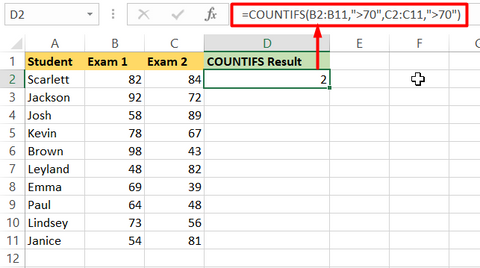### Example 3 - Using COUNTIFS to Count Cells With Values Between Two Numbers

You can also use COUNTIFS to calculate how many numbers lie between two numbers - a pretty neat little trick that avoids having to use multiple formulas or nesting.

In this example, we're looking at how many numbers are greater than 12 but less than 18. So here's the formula we would use to achieve that:

=COUNTIFS(B2:B8,”>12”,B2:B8,”<18”)

Here we're looking in the same range both times (B2:B8), but using the COUNTIFS function to match different criteria each time. In this case, greater than 12 and fewer than 18.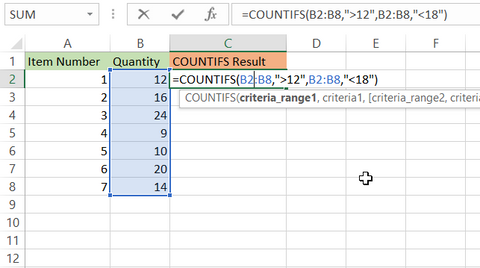That yields the result of 2 in this instance.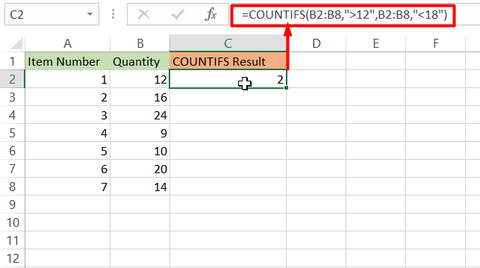### Example 4 - Using COUNTIFS When the Criteria Is a Cell Reference

Of course, you don’t have to embed your criteria into your formula when using COUNTIF. You can also reference a cell as your criteria.

Let's say that we have a dataset of student test results, and we want to show the number of students that have passed or failed that test. Simple enough. But what if we wanted to count how many students had passed but also achieved over a particular mark? We could deploy the COUNTIFS function to help us in this instance.

In this example, we've listed our two criteria in cells B13 and B14. In our formula, we refer to these and make them fixed references so we can drag the formula down.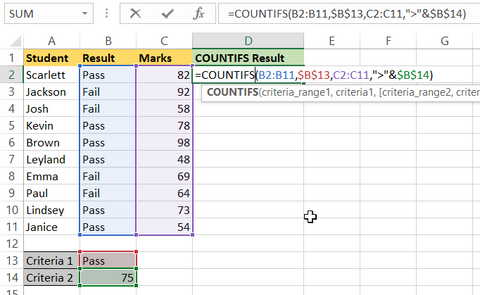Our result is 3.The added benefit of this is that we can now change Criteria 1 and 2 to be other values, and we’ll immediately see the new result.

## Important Notes About the COUNTIFS Function

We've just scratched the surface of what is possible with the impressive COUNTIFS function in Excel. There are so many different ways you can utilise COUNTIFS, especially when you start to think creatively about your criteria.

Here're a few things to be aware of when using COUNTIFS

• COUNTIFS is only available from Microsoft Excel 2000 onwards. If you can't find it when you type in =COUNTIFS, then your version doesn't have it!
• COUNTIFS is not case-sensitive.
• Every COUNTIFS formula must include at least one range.
• Each additional range in COUNTIFS must have the same number of rows and columns as the previous criteria and range.
• It is possible to use wildcards in your COUNTIFS criteria.
• The function allows both contiguous and non-contiguous ranges.
• Ensure there is a matching criterion for each range you select.
• When looking to count actual wildcards using COUNTIFS (a wildcard is * or ? for example), use a tilde (~) to achieve this.

## Why You'll Love COUNTIFS

The fact that COUNTIFS can handle multiple criteria compared to its famous cousin, COUNTIF, makes it a fantastic function in our opinion. Data analysis work you may have done in a Pivot Table could be done with a simple COUNTIFS formula instead.

It also removes the need for complicated nesting using multiple functions. In our eyes, it's a true super function!

## Frequently Asked Questions

#### 1. Can COUNTIFS have multiple Criteria?

Yes, COUNTIFS can have multiple criteria. For example, you can use multiple criteria in a COUNTIFS formula to calculate how many values sit between two values.

#### 2. How do you differentiate between COUNTIF and COUNTIFS?

The simplest difference between COUNTIF and COUNTIFS is that COUNTIF can count cells with a single condition from one range. COUNTIFS can do the same with multiple criteria and the same or different ranges.

## Get Started With COUNTIFS

Now, you understand what COUNTIFS is and what it does. Start practising with this function in real-life situations!

If this article got you excited about learning more Excel, we recommend getting started with these, free resources:

Alternatively, if it’s time you got a bit more serious about your spreadsheets, then an Excel course from Excel with Business might be for you.